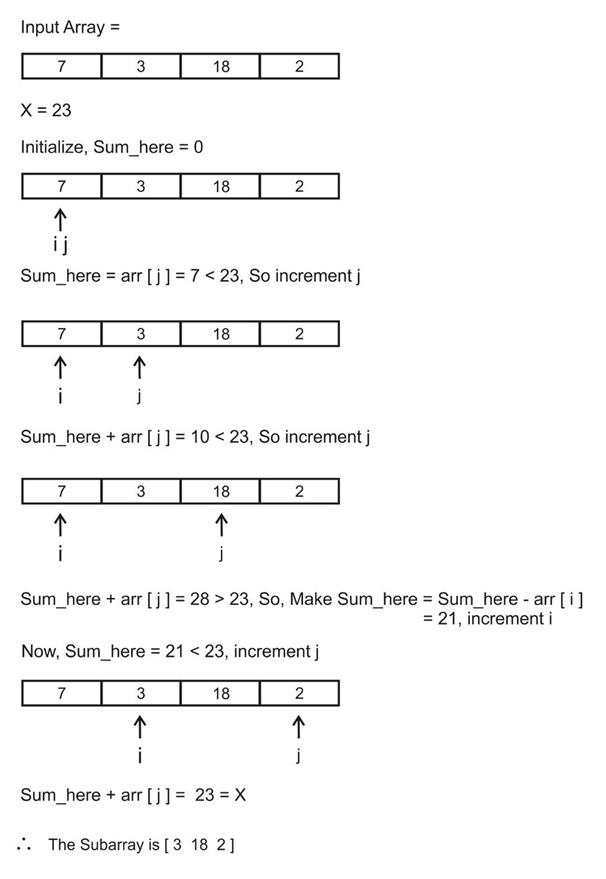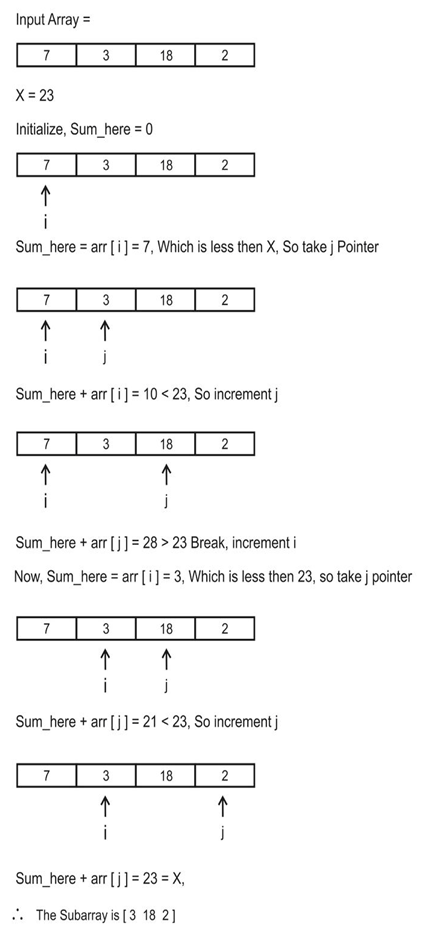# Find the subarray whose sum is equal to a given number X

### Example

INPUT
7, 3, 18, 2  and given number X = 23

OUTPUT
SUBARRAY IS 3, 18, 2 whose sum is 23

## ALGORITHM 1

TIME COMPLEXITY – O(N)
SPACE COMPLEXITY – O(1)

1.    We initialise two variables i and j such that both point to the starting index of an array. And take a variable sum_here and initialize it to 0.
2.    If the sum_here is less than X then add the element pointed by j  to variable sum_here and increment j.
3.    If the sum_here is greater than X then decrement sum_here by the value pointed by i and increment i.
4.    If the sum_here equals to X then we got the subarray starting with i and ending point is j.
5.    Else go to step 2.## C++ Program

```#include <bits/stdc++.h>
using namespace std;

int main()
{

int arr[] = {1,5,3,16,2,18,19};
int N = sizeof(arr)/sizeof(arr);

int X;
cin>>X; //the sum to which subarray sum should be equal

int i = 0 , j = 0;
int sum_here = 0;
while(i < N and j < N) //two pointer like variable to refer the subarray start and end
{
if(sum_here > X) //if sum exceeds then remove the starting element
{
sum_here -= arr[i++];
}

if(sum_here == X) //if the given sum is found
{
cout<<"Subarray sum is found\n";
for(int k=i;k<j;k++)
cout<<arr[k]<<" ";
return 0;
}

else if(sum_here < X)//if the sum is less than the given element then add the present element
sum_here += arr[j++];

}
if(sum_here == X)
{
cout<<"Subarray sum is found\n";
for(int k=i;k<j;k++)
cout<<arr[k]<<" ";
return 0;
}
else
cout<<"No subarray sum found with given X"<<endl;

return 0;
}```

## ALGORITHM 2

TIME COMPLEXITY – O(N2)
SPACE COMPLEXITY – O(1)

We simply run two loops.
1.    Select an element in the outer loop.
2.    Start from the next element and start adding them so as to obtain the subarray sum.
3.    If the sum is greater than X then break the inner loop and start with another subarray by incrementing i.
4.    If the sum is less than, add the element pointed by j.
5.    If the sum is equal to X then return the subarray pointed by i and j as start and end.## C++ Program

```#include <bits/stdc++.h>
using namespace std;

int main()
{
int arr[] = {1,5,3,16,2,18,19};
int N = sizeof(arr)/sizeof(arr);

int X;
cin>>X; //the sum to which subarray sum should be equal

for(int i=0;i<N;i++)	//select an element
{
int sum_here=arr[i];
if(sum_here == X) //if the element itself is the sum
{
cout<<"The sum is found \nThe subarray is :"<<arr[i]<<"\n";
return 0;
}

for(int j=i+1;j<N;j++)//check the subarrays whose sum is equal to X
{

if(sum_here + arr[j] == X)//if equal then subarray found
{
cout<<"The sum is found \nThe subarray is :\n";
for(int k=i;k<=j;k++)
cout <<arr[k]<<" ";
return 0;
}

else if(sum_here + arr[j] < X) //if less then add the current element
sum_here += arr[j];
else
break;
}
}

cout<<"No subarray sum found with given X"<<endl;
return 0;
}```

Translate »